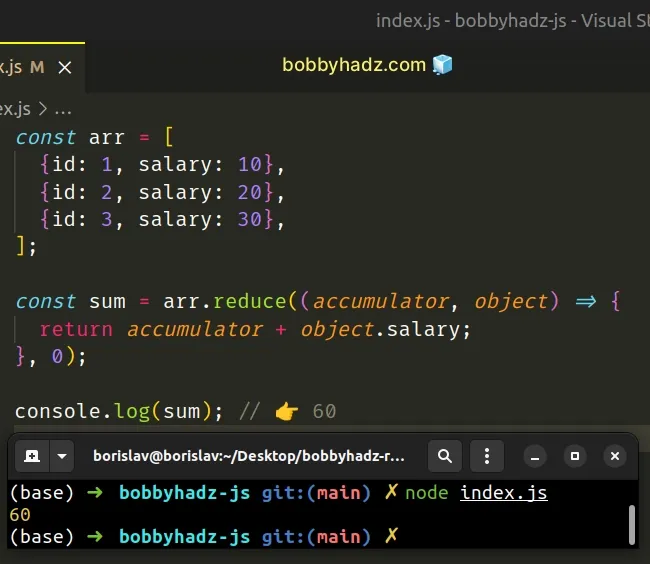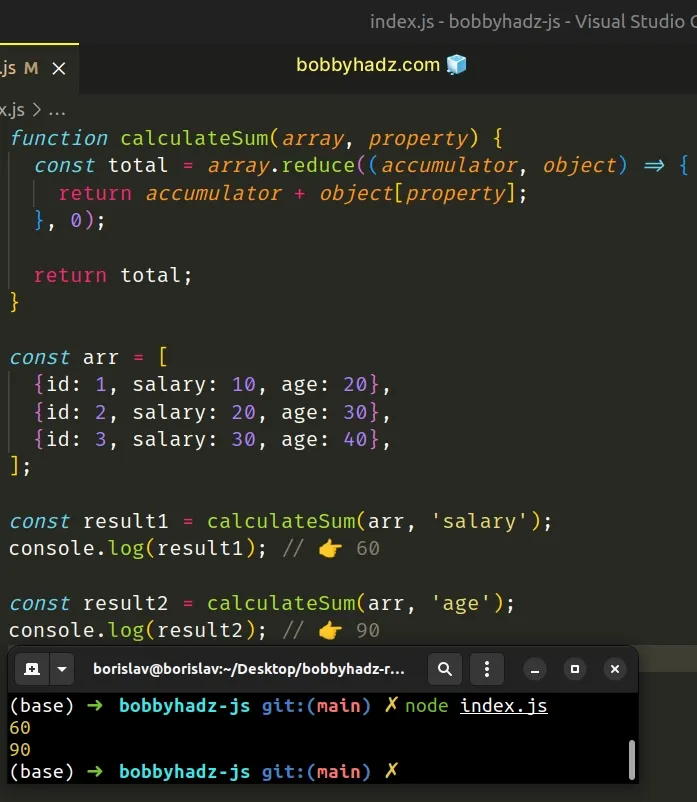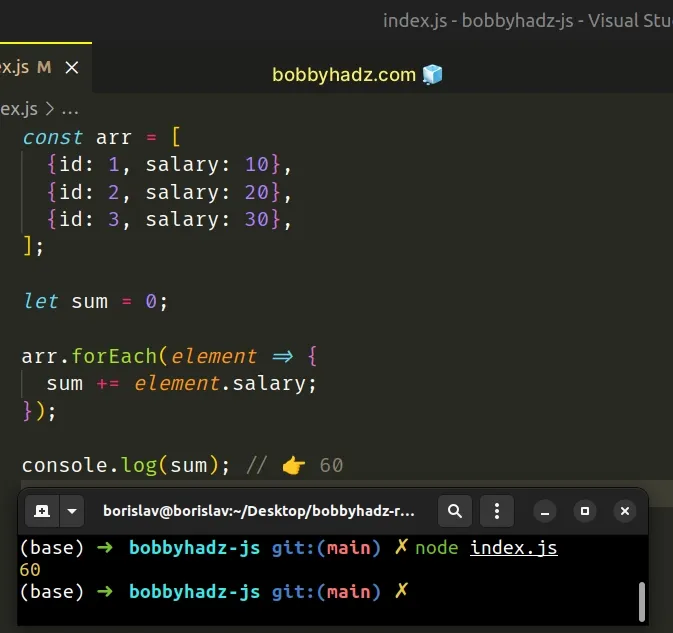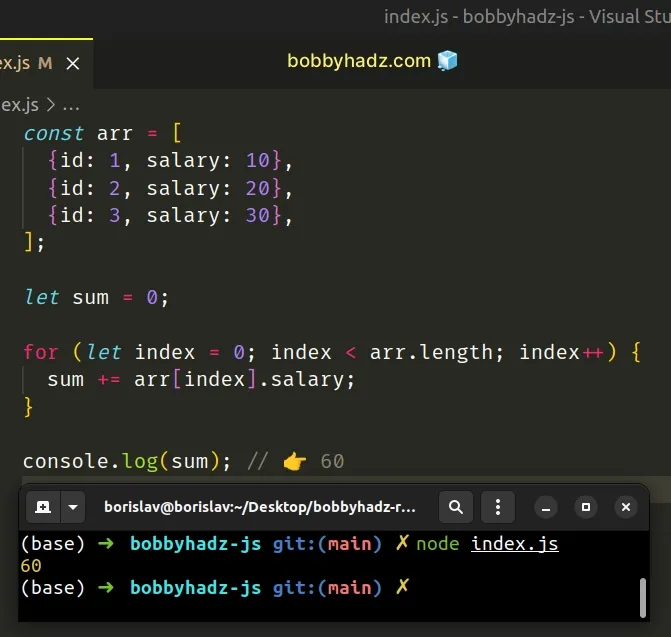# How to Sum a Property in an Array of Objects in JavaScriptLast updated: Dec 26, 2022
4 min## #Sum a Property in an Array of Objects in JavaScript

To sum a property in an array of objects:

1. Use the `reduce()` method to iterate over the array.
2. On each iteration increment the sum with the specific value.
3. The result will contain the sum of the values for the specific property.
index.js
```Copied!```const arr = [
{id: 1, salary: 10},
{id: 2, salary: 20},
{id: 3, salary: 30},
];

const sum = arr.reduce((accumulator, object) => {
return accumulator + object.salary;
}, 0);

console.log(sum); // 👉️ 60
``````The function we passed to the Array.reduce method gets invoked for each element (object) in the array.

The `accumulator` parameter gets an initial value of `0` because that's what we supplied as the second argument to the `reduce()` method.

We increment the `accumulator` by the value of the `salary` property for each object in the array.

The `reduce()` method returns the sum of the `salary` values of all objects in the array.

If you have to do this often, define a reusable function.

index.js
```Copied!```function calculateSum(array, property) {
const total = array.reduce((accumulator, object) => {
return accumulator + object[property];
}, 0);

}

const arr = [
{id: 1, salary: 10, age: 20},
{id: 2, salary: 20, age: 30},
{id: 3, salary: 30, age: 40},
];

const result1 = calculateSum(arr, 'salary');
console.log(result1); // 👉️ 60

const result2 = calculateSum(arr, 'age');
console.log(result2); // 👉️ 90
``````The `calculateSum` function takes an array and a property as parameters and calculates the sum of the specified property in the array of objects.

If you need to sum the values of an object, check out the following article.

An alternative and perhaps simpler approach is to use the `forEach` method.

## #Sum a Property in an Array of Objects using `Array.forEach()`

This is a three-step process:

1. Declare a `sum` variable using the `let` keyword and set it to `0`.
2. Call the `forEach()` method to iterate over the array.
3. On each iteration, increment the `sum` variable with the value of the object.
index.js
```Copied!```const arr = [
{id: 1, salary: 10},
{id: 2, salary: 20},
{id: 3, salary: 30},
];

let sum = 0;

arr.forEach(element => {
sum += element.salary;
});

console.log(sum); // 👉️ 60
``````The function we passed to the Array.forEach method gets called with each element (object) in the array.

Notice that we declared the `sum` variable using the `let` keyword. We can't reassign variables declared using `const`.

On each iteration of the loop, we increment the value stored in the `sum` variable to get the total amount.

This approach is more readable and intuitive, especially if you aren't used to how the `reduce()` method works.

If you have to do this often, define a reusable function.

index.js
```Copied!```function calculateSum(array, property) {
let sum = 0;

array.forEach(element => {
sum += element[property];
});

return sum;
}

const arr = [
{id: 1, salary: 10, age: 20},
{id: 2, salary: 20, age: 30},
{id: 3, salary: 30, age: 40},
];

const result1 = calculateSum(arr, 'salary');
console.log(result1); // 👉️ 60

const result2 = calculateSum(arr, 'age');
console.log(result2); // 👉️ 90
``````

The `calculateSum` function takes an array of objects and a property as parameters and calculates the sum of the specified property.

You can also achieve the same result by using a basic `for` loop.

## #Sum a Property in an Array of Objects using a `for` loop

This is a three-step process:

1. Declare a `sum` variable using the `let` keyword and set it to `0`.
2. Use a `for` loop to iterate over the array.
3. On each iteration, increment the `sum` variable with the value of the object.
index.js
```Copied!```const arr = [
{id: 1, salary: 10},
{id: 2, salary: 20},
{id: 3, salary: 30},
];

let sum = 0;

for (let index = 0; index < arr.length; index++) {
sum += arr[index].salary;
}

console.log(sum); // 👉️ 60
``````This code sample achieves the same result, however, instead of the `forEach()` method, we use a basic `for` loop.

Instead of accessing the element directly, we have to use the index of each iteration.

This is a bit indirect and cluttered, however, most developers are used to reading `for` loops.

You can also use a combination of the `Array.map()` and `Array.reduce()` methods to sum a property in an array of objects.

## #Sum a Property in an Array of Objects using `map()` and `reduce()`

This is a two-step process:

1. Use the `Array.map()` method to get an array of the values of the specific property.
2. Use the `Array.reduce()` method to sum the values in the array.
index.js
```Copied!```const arr = [
{id: 1, salary: 10},
{id: 2, salary: 20},
{id: 3, salary: 30},
];

const sum = arr
.map(obj => obj.salary)
.reduce((accumulator, current) => accumulator + current, 0);

console.log(sum); // 👉️ 60
``````

The function we passed to the `Array.map()` method gets called with each element (object) in the array.

On each iteration, we access the `salary` property and return its value.

index.js
```Copied!```const arr = [
{id: 1, salary: 10},
{id: 2, salary: 20},
{id: 3, salary: 30},
];

// 👇️ [ 10, 20, 30 ]
console.log(arr.map(obj => obj.salary));
``````

The `Array.map()` method returns a new array that only contains the values of the specified property.

The next step is to use the `Array.reduce()` method to calculate the sum of the values in the array.

index.js
```Copied!```const arr = [
{id: 1, salary: 10},
{id: 2, salary: 20},
{id: 3, salary: 30},
];

const sum = arr
.map(obj => obj.salary)
.reduce((accumulator, current) => accumulator + current, 0);

console.log(sum); // 👉️ 60
``````

We used `0` as the initial value of the `accumulator` variable.

Which approach you pick is a matter of personal preference. I'd use the `Array.forEach()` method or `Array.reduce()` as I find both options quite direct and easy to read.